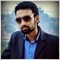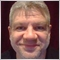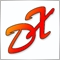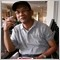# Lot Size Calculation from Risk Percentage88

i am trying to make an EA for auto risk calculation but still not successful.

i am trying to calculate risk from Stoploss value in pip.

Example my stoploss is 10pip & i want to take the risk of 5% of my total Account balance. it should calculate from these two parameters...

Here is my code i tried but not worked for me.

```//RiskCalculate
double riskcalculation(int Val_SL01)
{
AccRiskAmount = MathAbs(MathRound((MathMin(AccountEquity(),AccountBalance())/100)*RiskPercentage));
Symbol_RC = Symbol();
BaseCurr = StringSubstr(Symbol_RC,0,3);
QuoteCurr = StringSubstr(Symbol_RC,3,3);
AcctCurr = AccountCurrency();
_bid = MarketInfo(Symbol_RC,MODE_BID);
minlot = MarketInfo(Symbol_RC,MODE_MINLOT);
USDExchange = MarketInfo("EURUSD",MODE_BID);
lotSize = MarketInfo(Symbol_RC,MODE_LOTSIZE);
double ValPerPip;
//Calc By Pair Curruncy value per pip
if(Symbol()=="GDAXIm.lmx" && Symbol()=="XAUUSD.lmx"){ValPerPip=10;}
else{
if(QuoteCurr==AcctCurr){ ValPerPip = lotSize*tickSize;}
else if(BaseCurr==AcctCurr){ ValPerPip = (lotSize*tickSize)/_bid;}
else{ValPerPip = (lotSize*tickSize*USDExchange)/_bid;}
}
double lotsizecalc = NormalizeDouble(AccRiskAmount/(ValPerPip*Val_SL01),1);
if(lotsizecalc<minlot){lotsizecalc=minlot;}
return(NormalizeDouble(lotsizecalc,1));
}```

it would be really grateful if anyone have the code for the EA & help me out for the correct formula.Thanks43268

Shahzaib Idrees:

i am trying to make an EA for auto risk calculation but still not successful.

i am trying to calculate risk from Stoploss value in pip.

Example my stoploss is 10pip & i want to take the risk of 5% of my total Account balance. it should calculate from these two parameters...

Here is my code i tried but not worked for me.

it would be really grateful if anyone have the code for the EA & help me out for the correct formula.Thanks

Hello,

Please use the SRC button when you post code. Thank you.

This time, I edited it for you.43268

```   double tickSize      = SymbolInfoDouble(Symbol(),SYMBOL_TRADE_TICK_SIZE);
double valueToRisk   = risk / 100 * capital;
double tickCount     = sldistance / tickSize;
double lots          = valueToRisk / (tickCount * tickValue);```88

```   double tickSize      = SymbolInfoDouble(Symbol(),SYMBOL_TRADE_TICK_SIZE);
double valueToRisk   = risk / 100 * capital;
double tickCount     = sldistance / tickSize;```

i want to calculate risk from stoploss pips & risk perecentage value.[Deleted]
Shahzaib Idrees:

i want to calculate risk from stoploss pips & risk perecentage value.

https://www.mql5.com/ru/code/viewcode/14296/79100/lot_risk_sl__2.mq4Lot-SL propotion
• 2015.12.01
• Vasyl Nosal
• www.mql5.com
Функция возвращает размер лота, рассчитанный исходя из размера стоп-лосса и указанного в переменной риска.23717

try this..

https://www.mql5.com/en/code/13593Auto MM
• 2015.08.06
• Siti Latifah
• www.mql5.com61748

Siti Latifah:

try this..

https://www.mql5.com/en/code/1359343268

Shahzaib Idrees:

i want to calculate risk from stoploss pips & risk perecentage value.

I know, it's exactly the code I gave you.

I was supposing you can adapt the code yourself, not ?61748

Shahzaib Idrees:

i want to calculate risk from stoploss pips & risk perecentage value.

based on the code of Alain:

```   double tickSize      = SymbolInfoDouble(Symbol(),SYMBOL_TRADE_TICK_SIZE);
double valueToRisk   = risk / 100 * capital;
double tickCount     = sldistance / tickSize;
double lots          = valueToRisk / (tickCount * tickValue);
//--
double tickValueSize = tickValue * _Point / tickSize;
double stoploss      = valueToRisk  / (lots * tickValueSize) - spread;```88

Siti Latifah:

try this..

https://www.mql5.com/en/code/13593

Thanks @Siti...Really helpful for me :)88

Alain Verleyen:

I know, it's exactly the code I gave you.

I was supposing you can adapt the code yourself, not ?

yes i solved the issue i had. the only issue was to get the Exact PIP Value (1 PIP= How much \$).